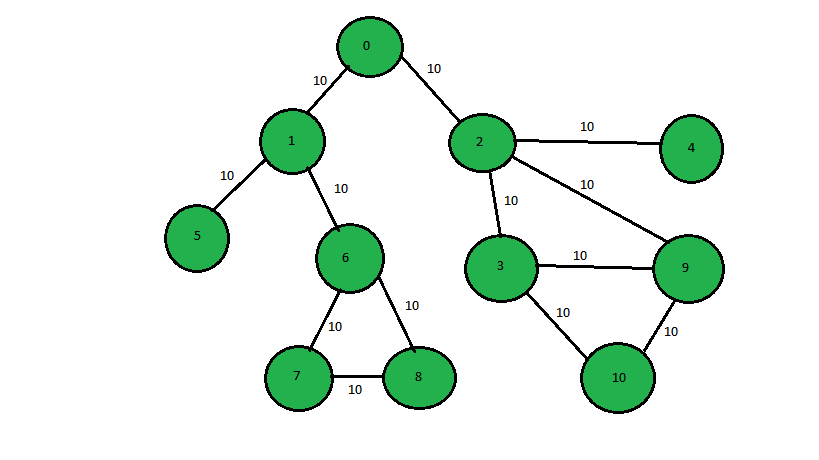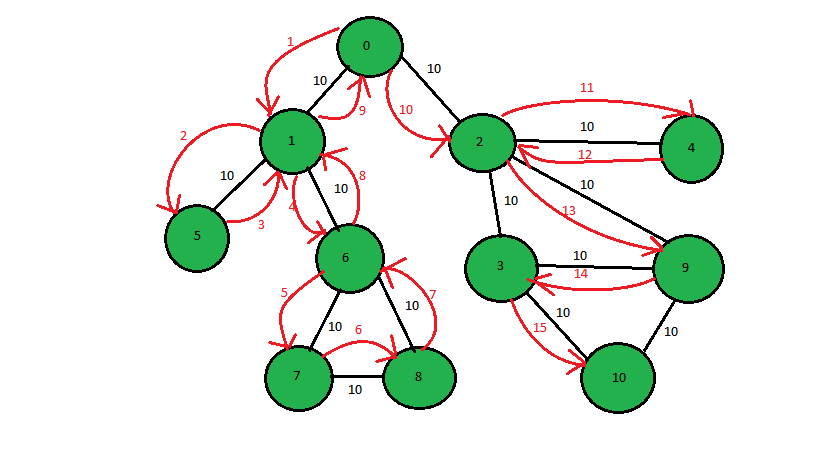# Print the DFS traversal step-wise (Backtracking also)

Given a graph, the task is to print the DFS traversal of a graph which includes the every step including the backtracking.```1st step:- 0 -> 1
2nd step:- 1 -> 5
3rd step:- 5 -> 1 (backtracking step)
4th step:- 1 -> 6...
and so on till all the nodes are visited.

Dfs step-wise(including backtracking) is:
0 1 5 1 6 7 8 7 6 1 0 2 4 2 9 3 10```

Note: In this above diagram the weight between the edges has just been added, it does not have any role in DFS-traversal## Recommended: Please try your approach on {IDE} first, before moving on to the solution.

Approach: DFS with Backtracking will be used here. First, visit every node using DFS simultaneously and keep track of the previously used edge and the parent node. If a node comes whose all the adjacent node has been visited, backtrack using the last used edge and print the nodes. Continue the steps and at every step, the parent node will become the present node. Continue the above steps to find the complete DFS traversal of the graph.

Below is the implementation of the above approach:

 `// C++ program to print the complete ` `// DFS-traversal of graph ` `// using back-tracking ` `#include ` `using` `namespace` `std; ` `const` `int` `N = 1000; ` `vector<``int``> adj[N]; ` ` `  `// Function to print the complete DFS-traversal ` `void` `dfsUtil(``int` `u, ``int` `node, ``bool` `visited[], ` `             ``vector > road_used, ``int` `parent, ``int` `it) ` `{ ` `    ``int` `c = 0; ` ` `  `    ``// Check if all th node is visited or not ` `    ``// and count unvisited nodes ` `    ``for` `(``int` `i = 0; i < node; i++) ` `        ``if` `(visited[i]) ` `            ``c++; ` ` `  `    ``// If all the node is visited return; ` `    ``if` `(c == node) ` `        ``return``; ` ` `  `    ``// Mark not visited node as visited ` `    ``visited[u] = ``true``; ` ` `  `    ``// Track the current edge ` `    ``road_used.push_back({ parent, u }); ` ` `  `    ``// Print the node ` `    ``cout << u << ``" "``; ` ` `  `    ``// Check for not visited node and proceed with it. ` `    ``for` `(``int` `x : adj[u]) { ` ` `  `        ``// call the DFs function if not visited ` `        ``if` `(!visited[x]) ` `            ``dfsUtil(x, node, visited, road_used, u, it + 1); ` `    ``} ` ` `  `    ``// Backtrack through the last ` `    ``// visited nodes ` `    ``for` `(``auto` `y : road_used) ` `        ``if` `(y.second == u) ` `            ``dfsUtil(y.first, node, visited, ` `                    ``road_used, u, it + 1); ` `} ` ` `  `// Function to call the DFS function ` `// which prints the DFS-travesal stepwise ` `void` `dfs(``int` `node) ` `{ ` ` `  `    ``// Create a array of visited ndoe ` `    ``bool` `visited[node]; ` ` `  `    ``// Vector to track last visited road ` `    ``vector > road_used; ` ` `  `    ``// Initialize all the node with false ` `    ``for` `(``int` `i = 0; i < node; i++) ` `        ``visited[i] = ``false``; ` ` `  `    ``// call the function ` `    ``dfsUtil(0, node, visited, road_used, -1, 0); ` `} ` ` `  `// Function to insert edges in Graph ` `void` `insertEdge(``int` `u, ``int` `v) ` `{ ` `    ``adj[u].push_back(v); ` `    ``adj[v].push_back(u); ` `} ` ` `  `// Driver Code ` `int` `main() ` `{ ` `    ``// number of nodes and edges in the graph ` `    ``int` `node = 11, edge = 13; ` ` `  `    ``// Function call to create the graph ` `    ``insertEdge(0, 1); ` `    ``insertEdge(0, 2); ` `    ``insertEdge(1, 5); ` `    ``insertEdge(1, 6); ` `    ``insertEdge(2, 4); ` `    ``insertEdge(2, 9); ` `    ``insertEdge(6, 7); ` `    ``insertEdge(6, 8); ` `    ``insertEdge(7, 8); ` `    ``insertEdge(2, 3); ` `    ``insertEdge(3, 9); ` `    ``insertEdge(3, 10); ` `    ``insertEdge(9, 10); ` ` `  `    ``// Call the function to print ` `    ``dfs(node); ` ` `  `    ``return` `0; ` `} `

Output:

```0 1 5 1 6 7 8 7 6 1 0 2 4 2 9 3 10
```

Attention reader! Don’t stop learning now. Get hold of all the important DSA concepts with the DSA Self Paced Course at a student-friendly price and become industry ready.

My Personal Notes arrow_drop_upCheck out this Author's contributed articles.

If you like GeeksforGeeks and would like to contribute, you can also write an article using contribute.geeksforgeeks.org or mail your article to contribute@geeksforgeeks.org. See your article appearing on the GeeksforGeeks main page and help other Geeks.

Please Improve this article if you find anything incorrect by clicking on the "Improve Article" button below.